True/False
Indicate whether the statement is true or false.

1.

The diagonals of a parallelogram bisect each other.

Multiple Choice
Identify the choice that best completes the statement or answers the question.

2.

The diagonals of the square with vertices at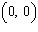,,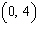, and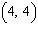meet at a point P. What are the coordinates of P?
 a.c.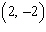b.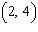d.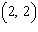3.In the parallelogram PQRS, which line segment is equal in length to QT?
 a. TS c. TR b. PT d. PQ

4.

A rectangle has vertices P, Q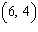, R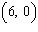, and S. What are the coordinates of the point of intersection of the diagonals PR and QS?
 a.c.b.d.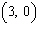5.

Which of the following quadrilaterals has diagonals of equal length?
 a. a kite c. a rhombus b. a parallelogram d. a square

6.

A quadrilateral has its vertices at A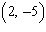, B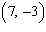, C, and D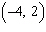. The line segment BC is parallel to which of these line segments?
 a. AB c. BD b. AD d. CD

7.

The sides of a parallelogram are given by the equations,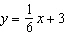,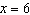, and.
Which of the following coordinates represents the point of intersection of the diagonals of the parallelogram?
 a.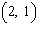c.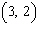b.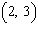d.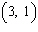Matching

Match the words or phrases with their definitions.
 a. angle bisectors f. concurrent b. centre g. congruent c. centroid h. diagonals d. chord i. isosceles e. collinear

8.

These meet at a point inside a triangle called the incentre.

9.

Lines that pass through the same point are called this.

10.

The medians of a triangle meet at a point inside the triangle with this name.

11.

Two of the medians of this kind of triangle are the same length.

12.

This is the name for a line segment drawn from one point on the circumference of a circle to another point on the circumference.

13.

In a parallelogram, these bisect each other.

14.

The perpendicular bisector of a chord passes through this part of a circle.

15.

Points that lie on the same line are called this.